# Selina Solutions Concise Mathematics Class 6 Chapter 15: Decimal Fractions Exercise 15(D)

Selina Solutions Concise Mathematics Class 6 Chapter 15 Decimal Fractions Exercise 15(D) mainly discusses the concept of conversion of paise to rupees, metre to centimetre, gram to kilogram and vice versa. The method of converting them and the steps to be followed are explained in an interactive manner for a better hold on the concepts, covered under this exercise. Students can improve time management skills by solving the exercise wise problems, with the help of solutions PDF. To obtain better conceptual knowledge, students can make use of Selina Solutions Concise Mathematics Class 6 Chapter 15 Decimal Fractions Exercise 15(D), PDF links which are available below

## Selina Solutions Concise Mathematics Class 6 Chapter 15: Decimal Fractions Exercise 15(D) Download PDF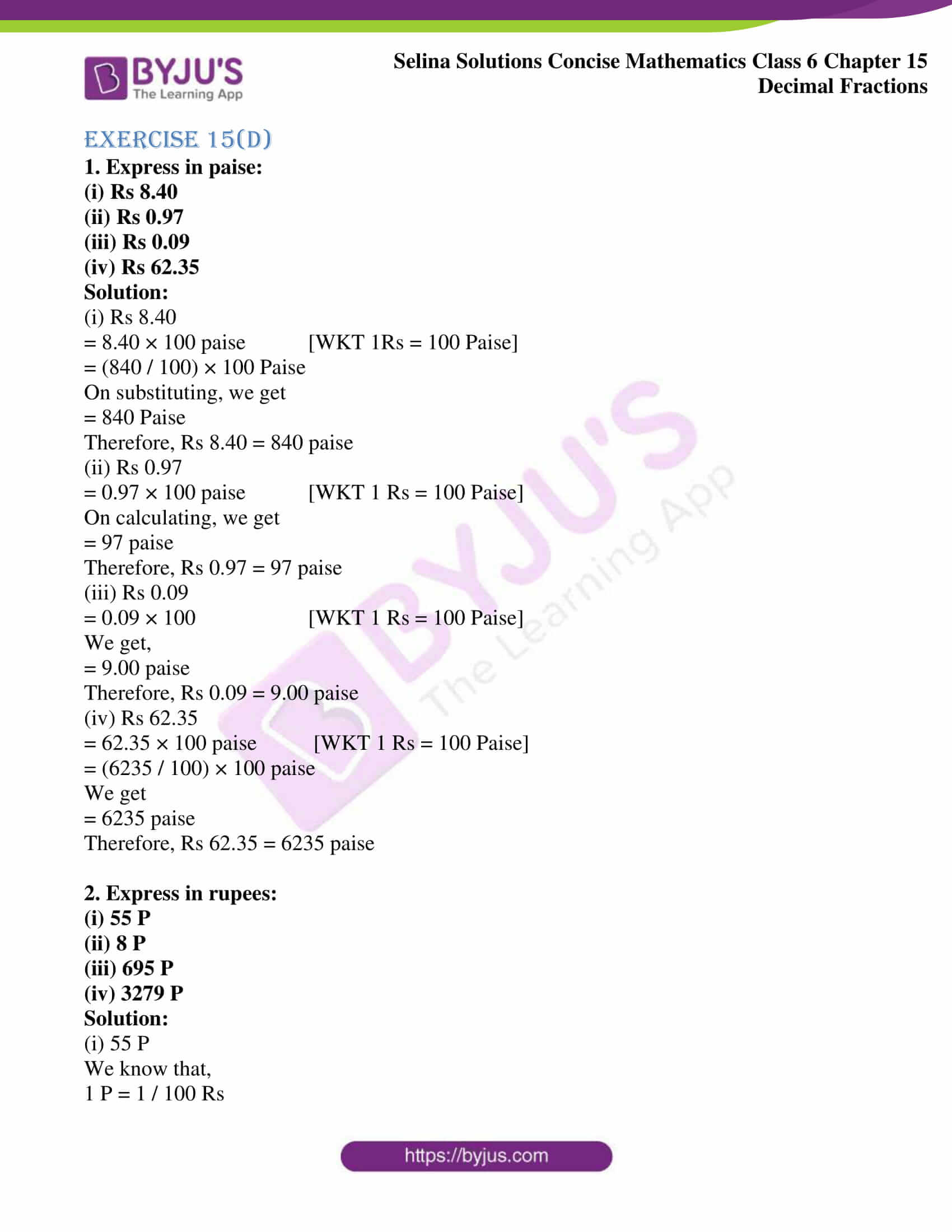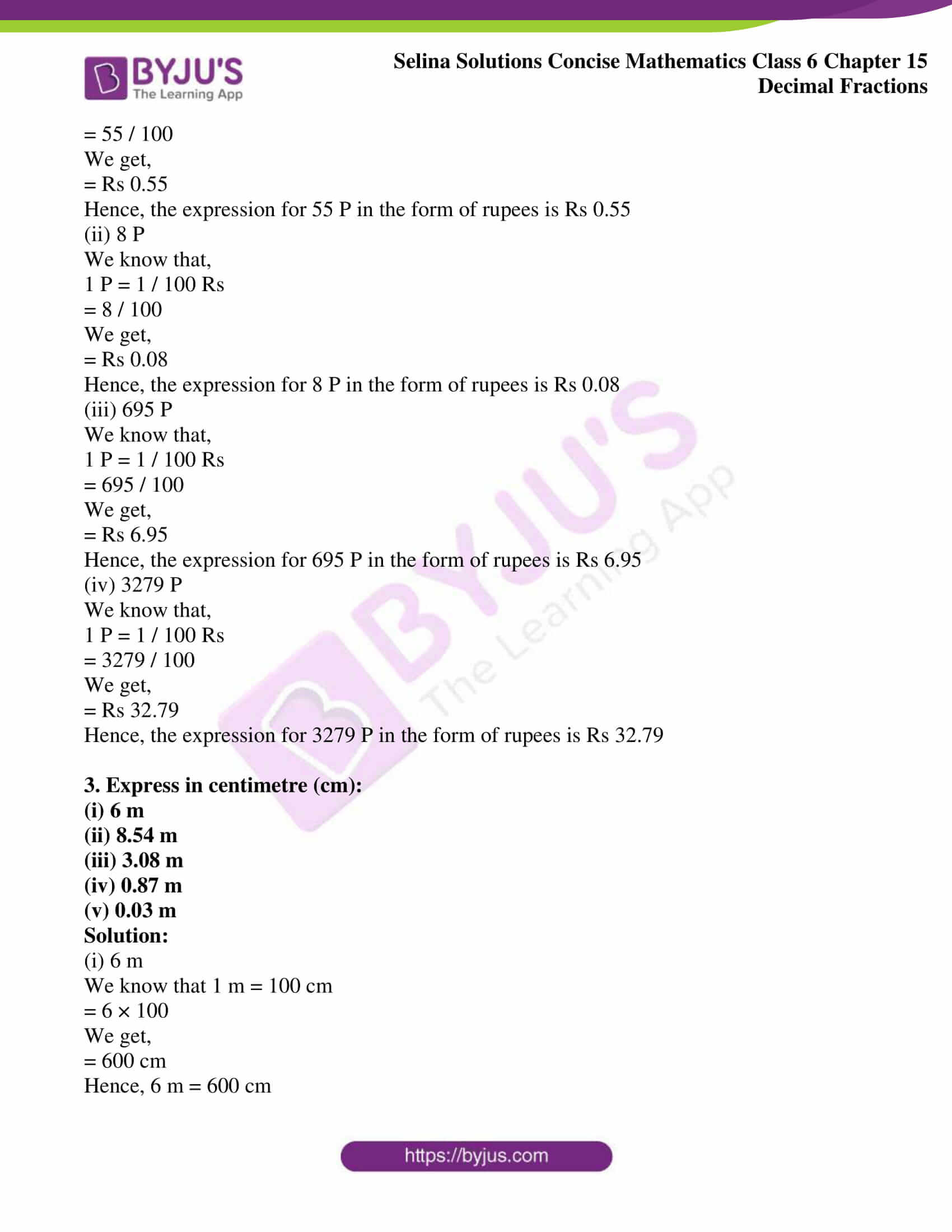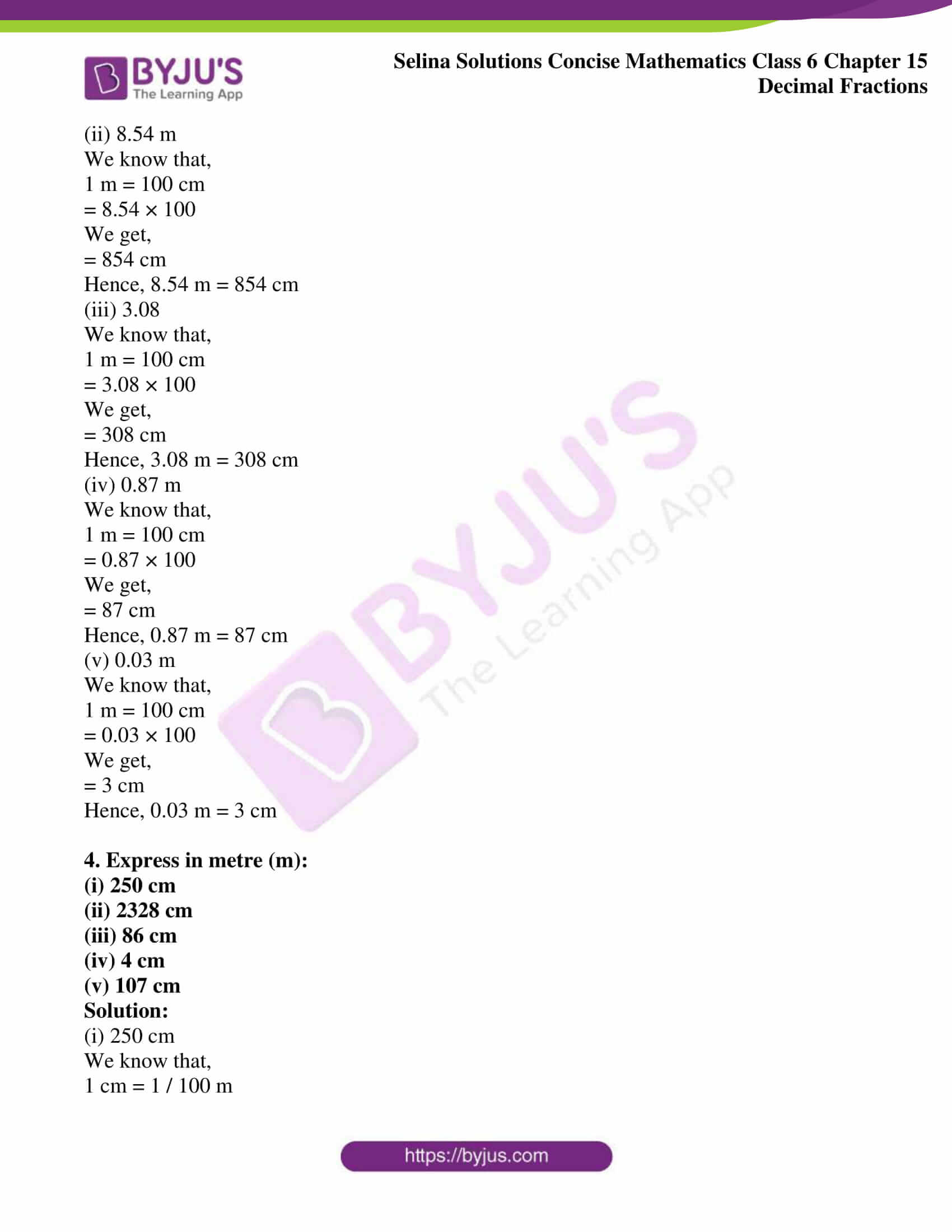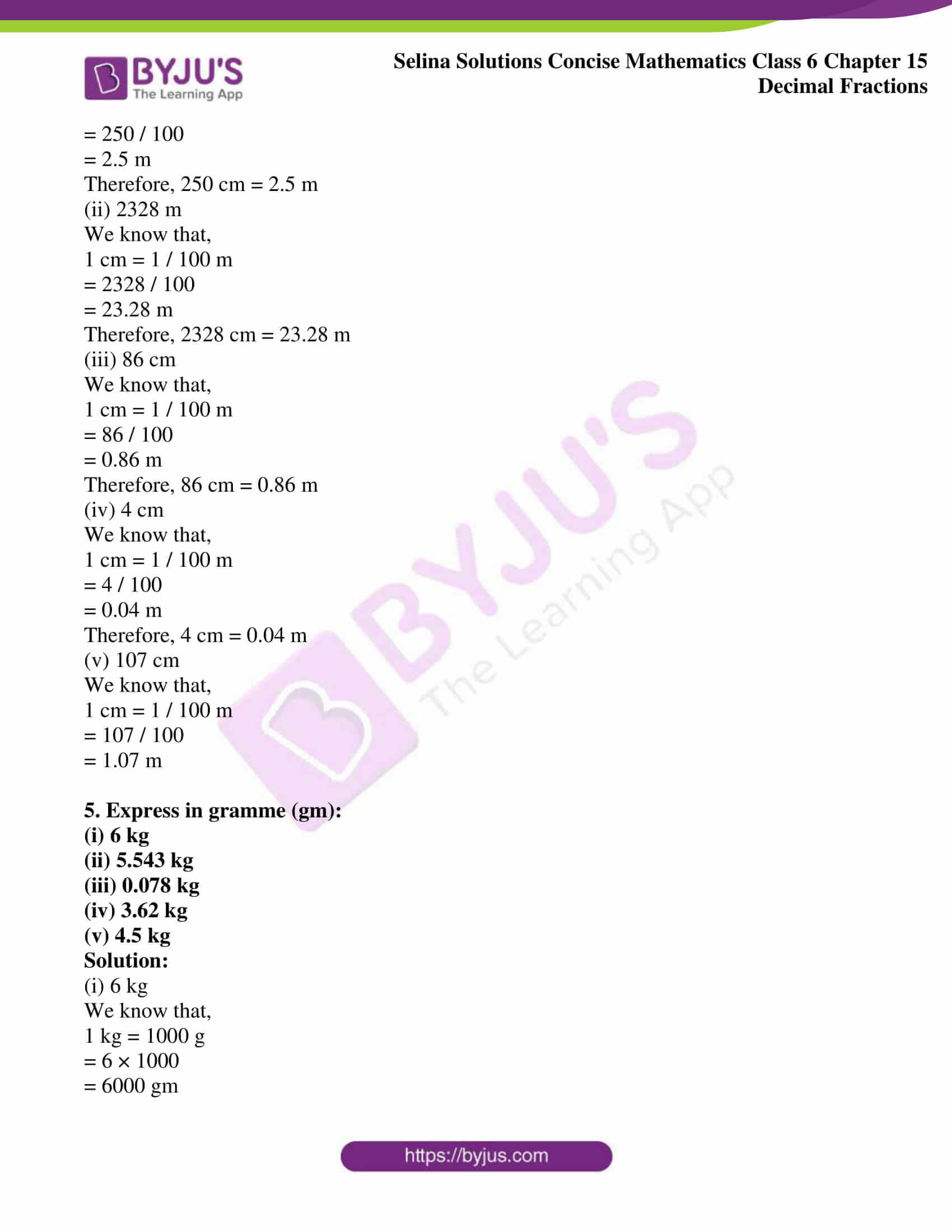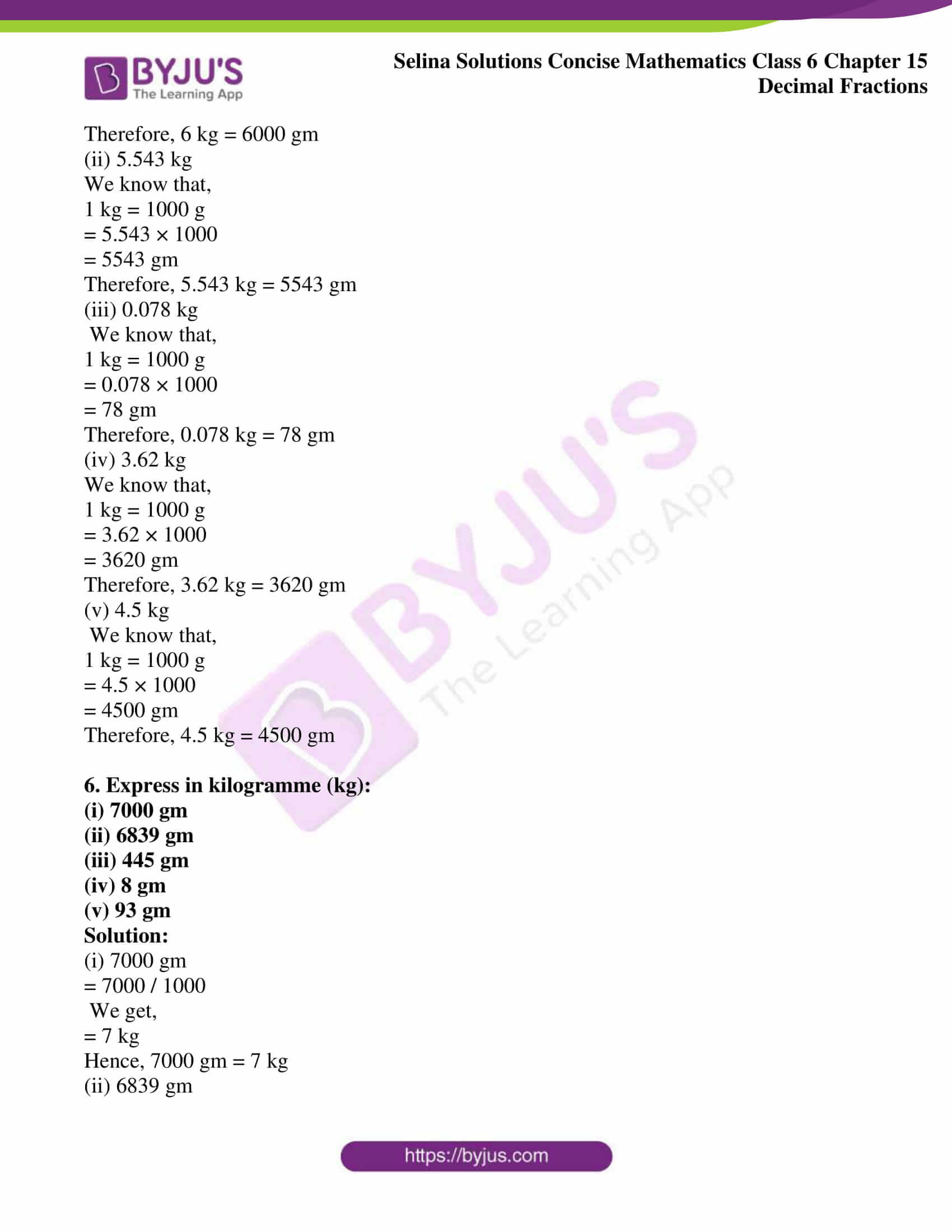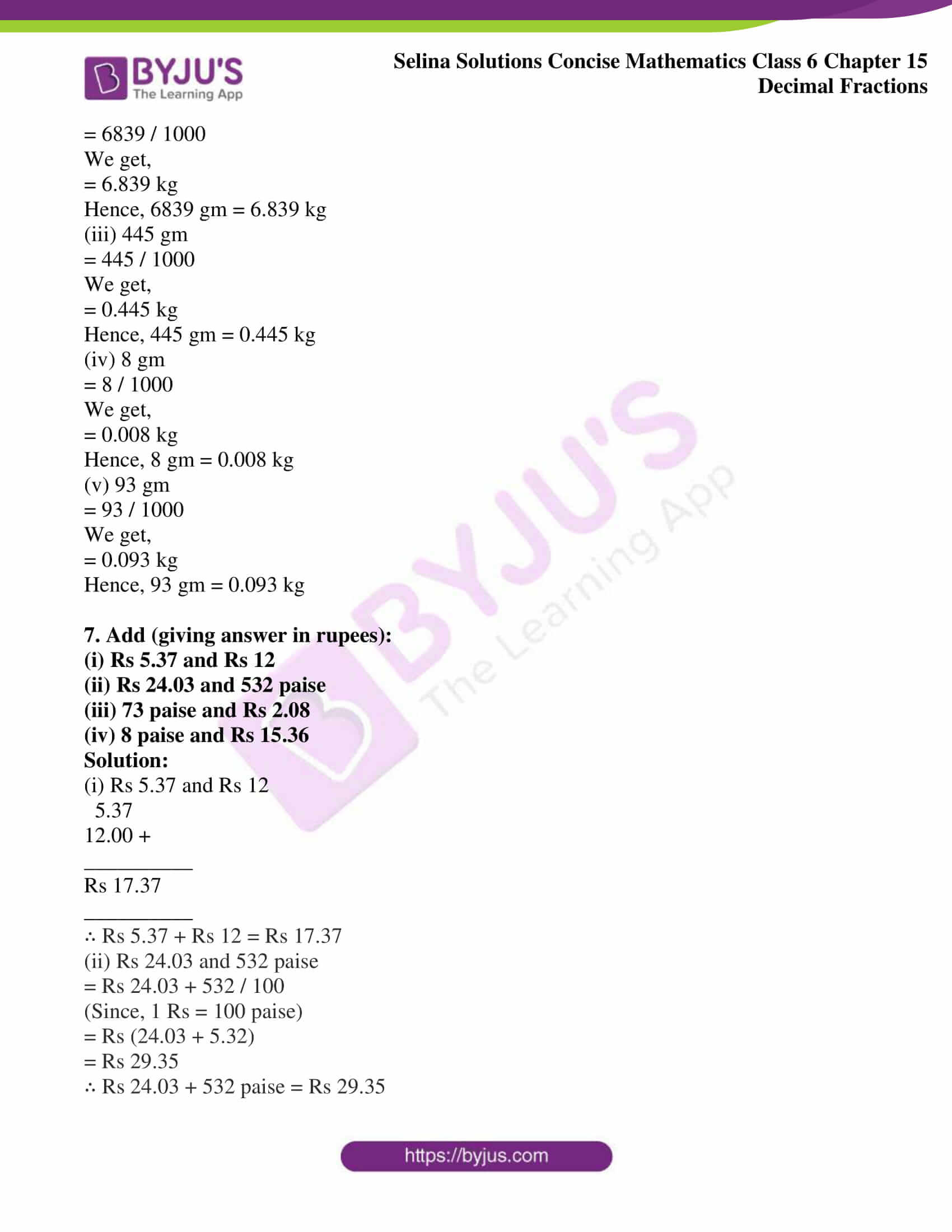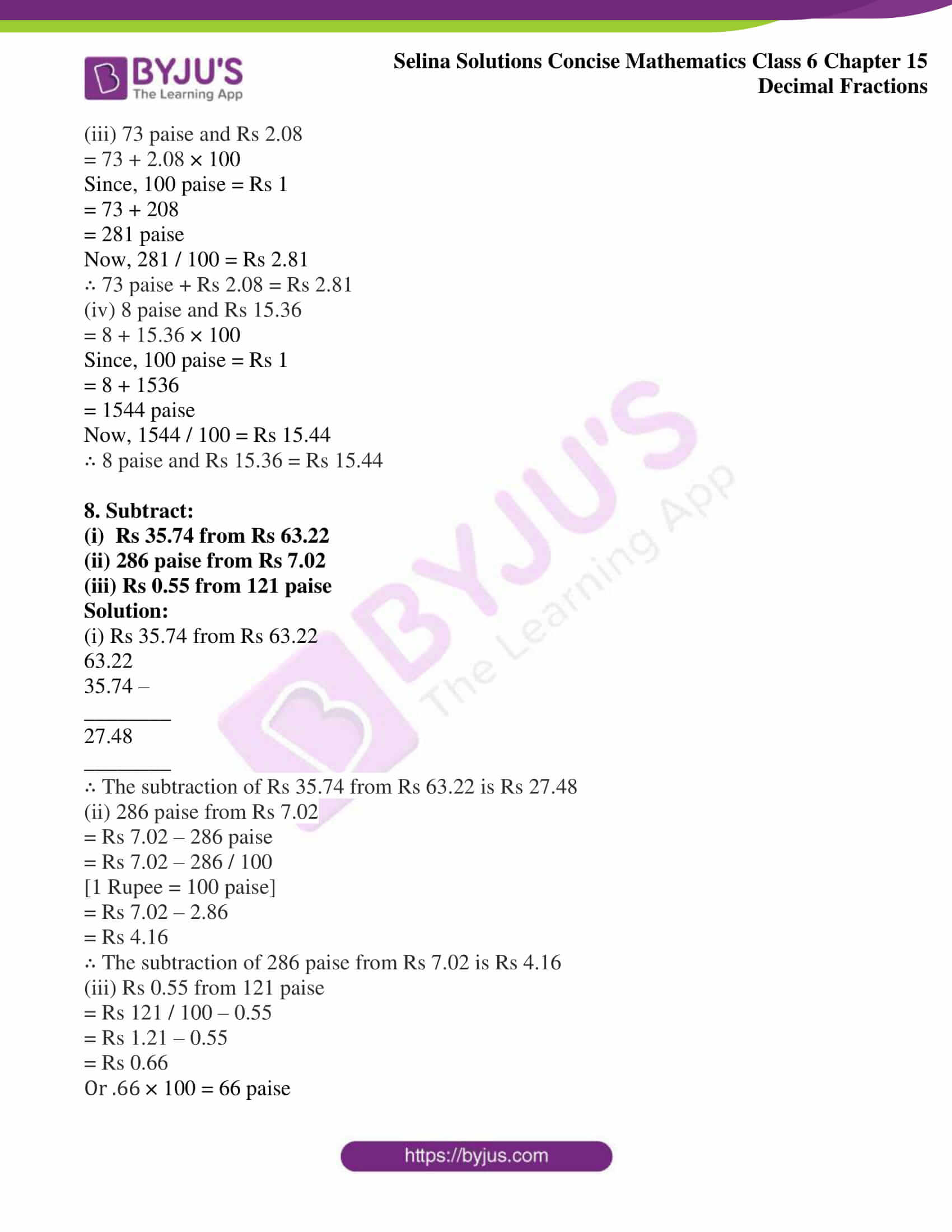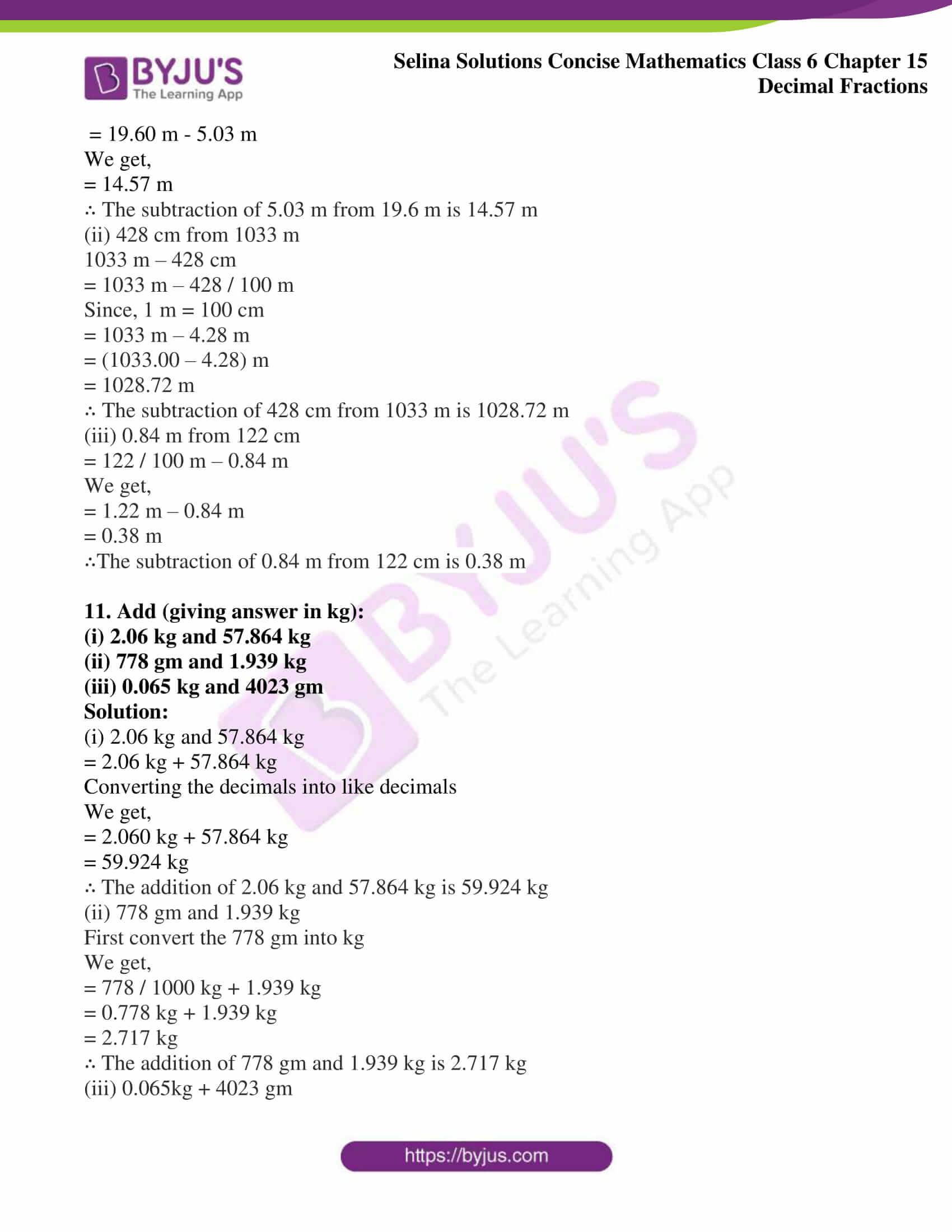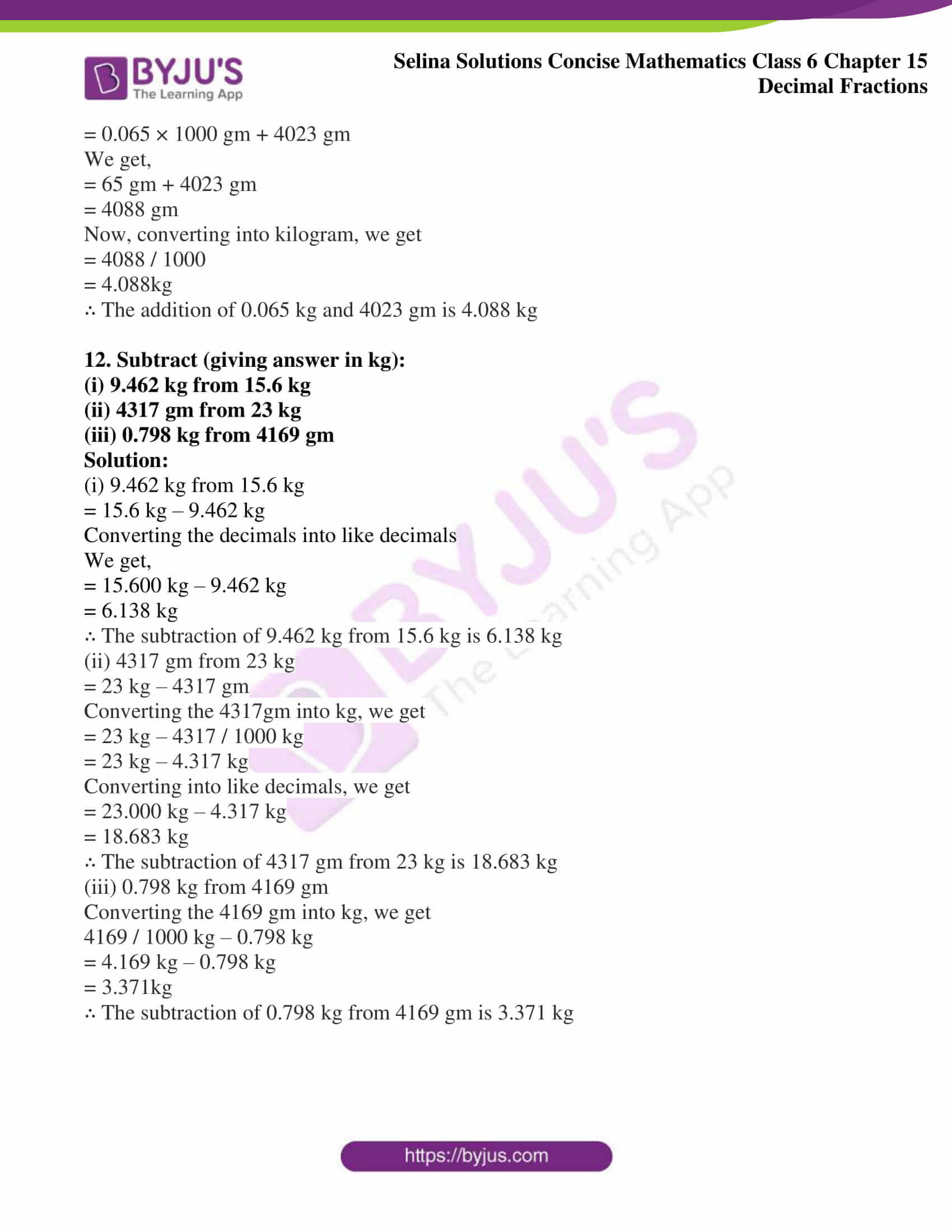### Access other exercises of Selina Solutions Concise Mathematics Class 6 Chapter 15: Decimal Fractions

Exercise 15(A) Solutions

Exercise 15(B) Solutions

Exercise 15(C) Solutions

Exercise 15(E) Solutions

### Access Selina Solutions Concise Mathematics Class 6 Chapter 15: Decimal Fractions Exercise 15(D)

Exercise 15(D)

1. Express in paise:

(i) Rs 8.40

(ii) Rs 0.97

(iii) Rs 0.09

(iv) Rs 62.35

Solution:

(i) Rs 8.40

= 8.40 × 100 paise [WKT 1Rs = 100 Paise]

= (840 / 100) × 100 Paise

On substituting, we get

= 840 Paise

Therefore, Rs 8.40 = 840 paise

(ii) Rs 0.97

= 0.97 × 100 paise [WKT 1 Rs = 100 Paise]

On calculating, we get

= 97 paise

Therefore, Rs 0.97 = 97 paise

(iii) Rs 0.09

= 0.09 × 100 [WKT 1 Rs = 100 Paise]

We get,

= 9.00 paise

Therefore, Rs 0.09 = 9.00 paise

(iv) Rs 62.35

= 62.35 × 100 paise [WKT 1 Rs = 100 Paise]

= (6235 / 100) × 100 paise

We get

= 6235 paise

Therefore, Rs 62.35 = 6235 paise

2. Express in rupees:

(i) 55 P

(ii) 8 P

(iii) 695 P

(iv) 3279 P

Solution:

(i) 55 P

We know that,

1 P = 1 / 100 Rs

= 55 / 100

We get,

= Rs 0.55

Hence, the expression for 55 P in the form of rupees is Rs 0.55

(ii) 8 P

We know that,

1 P = 1 / 100 Rs

= 8 / 100

We get,

= Rs 0.08

Hence, the expression for 8 P in the form of rupees is Rs 0.08

(iii) 695 P

We know that,

1 P = 1 / 100 Rs

= 695 / 100

We get,

= Rs 6.95

Hence, the expression for 695 P in the form of rupees is Rs 6.95

(iv) 3279 P

We know that,

1 P = 1 / 100 Rs

= 3279 / 100

We get,

= Rs 32.79

Hence, the expression for 3279 P in the form of rupees is Rs 32.79

3. Express in centimetre (cm):

(i) 6 m

(ii) 8.54 m

(iii) 3.08 m

(iv) 0.87 m

(v) 0.03 m

Solution:

(i) 6 m

We know that 1 m = 100 cm

= 6 × 100

We get,

= 600 cm

Hence, 6 m = 600 cm

(ii) 8.54 m

We know that,

1 m = 100 cm

= 8.54 × 100

We get,

= 854 cm

Hence, 8.54 m = 854 cm

(iii) 3.08

We know that,

1 m = 100 cm

= 3.08 × 100

We get,

= 308 cm

Hence, 3.08 m = 308 cm

(iv) 0.87 m

We know that,

1 m = 100 cm

= 0.87 × 100

We get,

= 87 cm

Hence, 0.87 m = 87 cm

(v) 0.03 m

We know that,

1 m = 100 cm

= 0.03 × 100

We get,

= 3 cm

Hence, 0.03 m = 3 cm

4. Express in metre (m):

(i) 250 cm

(ii) 2328 cm

(iii) 86 cm

(iv) 4 cm

(v) 107 cm

Solution:

(i) 250 cm

We know that,

1 cm = 1 / 100 m

= 250 / 100

= 2.5 m

Therefore, 250 cm = 2.5 m

(ii) 2328 m

We know that,

1 cm = 1 / 100 m

= 2328 / 100

= 23.28 m

Therefore, 2328 cm = 23.28 m

(iii) 86 cm

We know that,

1 cm = 1 / 100 m

= 86 / 100

= 0.86 m

Therefore, 86 cm = 0.86 m

(iv) 4 cm

We know that,

1 cm = 1 / 100 m

= 4 / 100

= 0.04 m

Therefore, 4 cm = 0.04 m

(v) 107 cm

We know that,

1 cm = 1 / 100 m

= 107 / 100

= 1.07 m

5. Express in gramme (gm):

(i) 6 kg

(ii) 5.543 kg

(iii) 0.078 kg

(iv) 3.62 kg

(v) 4.5 kg

Solution:

(i) 6 kg

We know that,

1 kg = 1000 g

= 6 × 1000

= 6000 gm

Therefore, 6 kg = 6000 gm

(ii) 5.543 kg

We know that,

1 kg = 1000 g

= 5.543 × 1000

= 5543 gm

Therefore, 5.543 kg = 5543 gm

(iii) 0.078 kg

We know that,

1 kg = 1000 g

= 0.078 × 1000

= 78 gm

Therefore, 0.078 kg = 78 gm

(iv) 3.62 kg

We know that,

1 kg = 1000 g

= 3.62 × 1000

= 3620 gm

Therefore, 3.62 kg = 3620 gm

(v) 4.5 kg

We know that,

1 kg = 1000 g

= 4.5 × 1000

= 4500 gm

Therefore, 4.5 kg = 4500 gm

6. Express in kilogramme (kg):

(i) 7000 gm

(ii) 6839 gm

(iii) 445 gm

(iv) 8 gm

(v) 93 gm

Solution:

(i) 7000 gm

= 7000 / 1000

We get,

= 7 kg

Hence, 7000 gm = 7 kg

(ii) 6839 gm

= 6839 / 1000

We get,

= 6.839 kg

Hence, 6839 gm = 6.839 kg

(iii) 445 gm

= 445 / 1000

We get,

= 0.445 kg

Hence, 445 gm = 0.445 kg

(iv) 8 gm

= 8 / 1000

We get,

= 0.008 kg

Hence, 8 gm = 0.008 kg

(v) 93 gm

= 93 / 1000

We get,

= 0.093 kg

Hence, 93 gm = 0.093 kg

(i) Rs 5.37 and Rs 12

(ii) Rs 24.03 and 532 paise

(iii) 73 paise and Rs 2.08

(iv) 8 paise and Rs 15.36

Solution:

(i) Rs 5.37 and Rs 12

5.37

12.00 +

__________

Rs 17.37

__________

∴ Rs 5.37 + Rs 12 = Rs 17.37

(ii) Rs 24.03 and 532 paise

= Rs 24.03 + 532 / 100

(Since, 1 Rs = 100 paise)

= Rs (24.03 + 5.32)

= Rs 29.35

∴ Rs 24.03 + 532 paise = Rs 29.35

(iii) 73 paise and Rs 2.08

= 73 + 2.08 × 100

Since, 100 paise = Rs 1

= 73 + 208

= 281 paise

Now, 281 / 100 = Rs 2.81

∴ 73 paise + Rs 2.08 = Rs 2.81

(iv) 8 paise and Rs 15.36

= 8 + 15.36 × 100

Since, 100 paise = Rs 1

= 8 + 1536

= 1544 paise

Now, 1544 / 100 = Rs 15.44

∴ 8 paise and Rs 15.36 = Rs 15.44

8. Subtract:

(i) Rs 35.74 from Rs 63.22

(ii) 286 paise from Rs 7.02

(iii) Rs 0.55 from 121 paise

Solution:

(i) Rs 35.74 from Rs 63.22

63.22

35.74 –

________

27.48

________

∴ The subtraction of Rs 35.74 from Rs 63.22 is Rs 27.48

(ii) 286 paise from Rs 7.02

= Rs 7.02 – 286 paise

= Rs 7.02 – 286 / 100

[1 Rupee = 100 paise]

= Rs 7.02 – 2.86

= Rs 4.16

∴ The subtraction of 286 paise from Rs 7.02 is Rs 4.16

(iii) Rs 0.55 from 121 paise

= Rs 121 / 100 – 0.55

= Rs 1.21 – 0.55

= Rs 0.66

Or .66 × 100 = 66 paise

∴ The subtraction of Rs 0.55 from 121 paise = 66 paise

(i) 2.4 m and 1.78 m

(ii) 848 cm and 2.9 m

(iii) 0.93 m and 64 cm

Solution:

(i) 2.4 m and 1.78 m

2.40 m

1.78 m +

_________

4.18 m

_________

∴ The addition of 2.4 m and 1.78 m = 4.18 m

(ii) 848 cm and 2.9 m

= 848 / 100 m + 2.9 m {1 m = 100 cm}

= 8.48 m + 2.9 m

8.48

2.9 +

___________

11.38

____________

∴ The addition of 848 cm and 2.9 m = 11.38 m

(iii) 0.93 m + 64 cm

= 0.93 m + 64 / 100 cm

= 0.93 m + 0.64 m

0.93

0.64 +

___________

1.57

____________

∴ The addition of 0.93 m + 64 cm = 1.57 m

10. Subtract (giving answer in metre):

(i) 5.03 m from 19.6 m

(ii) 428 cm from 1033 m

(iii) 0.84 m from 122 cm

Solution:

(i) 5.03 m from 19.6 m

= 19.60 m – 5.03 m

We get,

= 14.57 m

∴ The subtraction of 5.03 m from 19.6 m is 14.57 m

(ii) 428 cm from 1033 m

1033 m – 428 cm

= 1033 m – 428 / 100 m

Since, 1 m = 100 cm

= 1033 m – 4.28 m

= (1033.00 – 4.28) m

= 1028.72 m

∴ The subtraction of 428 cm from 1033 m is 1028.72 m

(iii) 0.84 m from 122 cm

= 122 / 100 m – 0.84 m

We get,

= 1.22 m – 0.84 m

= 0.38 m

∴The subtraction of 0.84 m from 122 cm is 0.38 m

(i) 2.06 kg and 57.864 kg

(ii) 778 gm and 1.939 kg

(iii) 0.065 kg and 4023 gm

Solution:

(i) 2.06 kg and 57.864 kg

= 2.06 kg + 57.864 kg

Converting the decimals into like decimals

We get,

= 2.060 kg + 57.864 kg

= 59.924 kg

∴ The addition of 2.06 kg and 57.864 kg is 59.924 kg

(ii) 778 gm and 1.939 kg

First convert the 778 gm into kg

We get,

= 778 / 1000 kg + 1.939 kg

= 0.778 kg + 1.939 kg

= 2.717 kg

∴ The addition of 778 gm and 1.939 kg is 2.717 kg

(iii) 0.065kg + 4023 gm

= 0.065 × 1000 gm + 4023 gm

We get,

= 65 gm + 4023 gm

= 4088 gm

Now, converting into kilogram, we get

= 4088 / 1000

= 4.088kg

∴ The addition of 0.065 kg and 4023 gm is 4.088 kg

12. Subtract (giving answer in kg):

(i) 9.462 kg from 15.6 kg

(ii) 4317 gm from 23 kg

(iii) 0.798 kg from 4169 gm

Solution:

(i) 9.462 kg from 15.6 kg

= 15.6 kg – 9.462 kg

Converting the decimals into like decimals

We get,

= 15.600 kg – 9.462 kg

= 6.138 kg

∴ The subtraction of 9.462 kg from 15.6 kg is 6.138 kg

(ii) 4317 gm from 23 kg

= 23 kg – 4317 gm

Converting the 4317gm into kg, we get

= 23 kg – 4317 / 1000 kg

= 23 kg – 4.317 kg

Converting into like decimals, we get

= 23.000 kg – 4.317 kg

= 18.683 kg

∴ The subtraction of 4317 gm from 23 kg is 18.683 kg

(iii) 0.798 kg from 4169 gm

Converting the 4169 gm into kg, we get

4169 / 1000 kg – 0.798 kg

= 4.169 kg – 0.798 kg

= 3.371kg

∴ The subtraction of 0.798 kg from 4169 gm is 3.371 kg